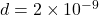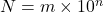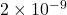## DNA’s diameter is 0.000000002 meters what is the measurement in scientific notation

Question

DNA’s diameter is 0.000000002 meters what is the measurement in scientific notation

in progress 0
2 months 2021-07-28T01:12:33+00:00 1 Answers 1 views 0Explanation:

It is given that, DNA’s diameter is 0.000000002 meters. We need to convert the diameter into scientific notation.

Any number can be written in the form of scientific notation as follows :Where, m is a real no and n is any integer

We have, d = 0.000000002 m

There are 8 zeroes before 2. We can shift the decimal after 2 so as to write it into scientific notation.

So,Hence, the diameter of the DNA is.